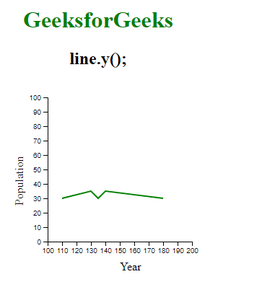# D3.js line.y() Function

The line.y() function is used to set the y accessor to the function or to a number and then returns this to the line generator function invoked by d3.line() function. If y is not specified then it returns the current y accessor.

Approach: In this example to create the line chart, first of all, create the SVG element and set its width and height as in the below example. Then create a CSV file, the data of the file is given in the example. We append the group container to the SVG. After this create a line generator function using d3.line() function.

After creating the line generator function set the x-scale and y-scale domain and range and append the scale to the SVG. Then give the x-scale data and y-scale data using line.x() and line.y() to the x-axis and y-axis. After this append the path to SVG and give the data from the line generator function to the “d” attribute of the path container. Give some style to the line chart and its done.

Syntax:

`line.y([y]);`

Parameters: This function accepts a single parameter as mentioned above and described below.

• y: This parameter is used to set the y-accessor.

Return Values: This function does not return anything.

Below given are a few examples of the function given above.

Example:

 `  ` `<``html` `lang``=``"en"``>  ` `    ``<``head``>  ` `        ``<``meta` `charset``=``"UTF-8"` `/>  ` `        ``<``meta` `            ``property``=``"viewport"` `            ``content="``width``=``device``-width,  ` `                    ``initial-scale``=``1``.0"/>  ` `        ``<``title``>GeeksforGeeks  ` `        ``` `        ``<``script` `src``=  ` `        ``"https://d3js.org/d3.v4.min.js"``>  ` `        `` ` `    `` ` `    ``<``body``>  ` `        ``<``div` `style``=``"width:300px; height:300px;"``> ` `            ``<``center``> ` `                ``<``h1` `style``=``"color:green"``> ` `                    ``GeeksforGeeks ` `                ``  ` `                ``<``h2``> ` `                    ``line.y(); ` `                ``  ` `            `` ` `            ``<``svg` `width``=``"300"` `height``=``"300"``> ` `            `` ` `        `` ` `        ``<``script``> ` `        ``// Selecting SVG and appending group tag ` `        ``var svg = d3.select("svg") ` `          ``.append("g") ` `            ``.attr("transform", ` `                  ``"translate(80, -80)"); ` `        ``// Data for the CSV file ` `            ``// year, population ` `            ``// 110, 20 ` `            ``// 130, 25 ` `            ``// 135, 20 ` `            ``// 140, 25 ` `            ``// 180, 20 ` ` `  `        ``// Fetching data from CSV file ` `        ``d3.csv("data.csv", ` `          ``(data)=>{ ` `            ``// Setting domain and range of x-scale  ` `            ``var x = d3.scaleLinear() ` `              ``.domain([100, 200]) ` `              ``.range([ 0, 200]); ` ` `  `            ``// Appending x-axis ` `            ``svg.append("g") ` `              ``.attr("transform", "translate(0, 300)") ` `              ``.call(d3.axisBottom(x)); ` `              ``svg.append("text")              ` `                ``.attr("transform", "translate(100, 340)") ` `                ``.text("Year"); ` `         `  `            ``// Setting domain and range of y-scale ` `            ``var y = d3.scaleLinear() ` `              ``.domain([0, 100]) ` `              ``.range([ 200, 0 ]); ` ` `  `            ``// Appending y-axis ` `            ``svg.append("g") ` `              ``.attr("transform", "translate(0, 100)") ` `              ``.call(d3.axisLeft(y)); ` ` `  `            ``svg.append("text")              ` `            ``.attr("transform", "rotate(-90)") ` `            ``.attr("y", -50) ` `            ``.attr("x", -250) ` `            ``.attr("dy", "1em") ` `            ``.text("Population"); ` `                 `  `         `  `            ``// Constructing line generator ` `            ``var line=d3.line(); ` `            ``line.x(function(d) { return x(+d.year) }); ` ` `  `            ``// Using line.x() Function ` `            ``line.y(function(d) { return y(+d.population) }); ` ` `  `            ``// Appending the path i.e the line ` `            ``svg.append("path") ` `              ``.datum(data) ` `              ``.attr("stroke", "green") ` `              ``.attr("stroke-width", "2") ` `              ``.attr("fill", "none") ` `              ``.attr("d", line) ` `              ``.attr("transform", "translate(0, 80)"); ` `        ``}); ` `        ``  ` `    ``  ` ` `

Output:Whether you're preparing for your first job interview or aiming to upskill in this ever-evolving tech landscape, GeeksforGeeks Courses are your key to success. We provide top-quality content at affordable prices, all geared towards accelerating your growth in a time-bound manner. Join the millions we've already empowered, and we're here to do the same for you. Don't miss out - check it out now!

Previous
Next